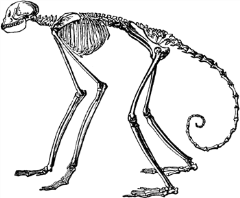Here is a little experiment. I have built a bunch of programmed agents that are using a variety of strategies to try and predict the outcome of a randomized event. The event in question is the roll of a dice. The twist is that the probabilities of all sides of the dice are not equal: there is a distribution of probabilities that is itself decided randomly before the experiment.

Here is a table showing how well each of the agents did on a hundred throws of that unfair dice:

• Agent 1: 18
• Agent 2: 22
• Agent 3: 20
• Agent 4: 10
• Agent 5: 14

Assuming you can't make any further tests, which agent would you hire to predict future throws?

The correct answer is none of them: you just don't have enough data. So let's throw the dice an additional thousand times:

• Agent 1: 180
• Agent 2: 188
• Agent 3: 176
• Agent 4: 136
• Agent 5: 168

What can we notice here? Agent 2 still seems to be doing pretty well, and agent 4 is still doing poorly, might you say. Well, that's true but irrelevant.

What you should be noticing is that the average probability of a hit over all the agents and over all the throws we've made is 0.169. But wait a minute. The probability of a completely random number between 1 and 6 is 0.167. That's pretty close. Just way too close to be a coincidence, as additional data would confirm.

You should by now have understood that I lied: that dice is not weighed at all, the numbers are as close to random as I know how to make (I used crypto-random numbers).

Our five agents do have various strategies (ranging from always picking the same number to picking the number that came out most often in the past) but the point is that it doesn't matter. There is no way to predict a random phenomenon (otherwise it's not random). No strategy works. None ever will. They are all equivalent to chance.

Now what am I getting at? The main lessons we can extract from this are the following:

1. You can usually determine with a good level of certainty whether a phenomenon is random by confronting its statistics with something you know to be random (it's sometimes trickier than that but is mostly reliable).
2. Luck in the past is not an indicator of luck in the future. Do not reward it.
3. In order to distinguish luck from talent, you need to determine first whether the domain where they apply is predictable and only once that's been shown, to consider previous results of the candidate.

To conclude, I'll leave you with this thought. What profession rewards its members with extravagant bonuses whereas it's been shown that dart-throwing monkeys were consistently doing better than any of them in the long run?